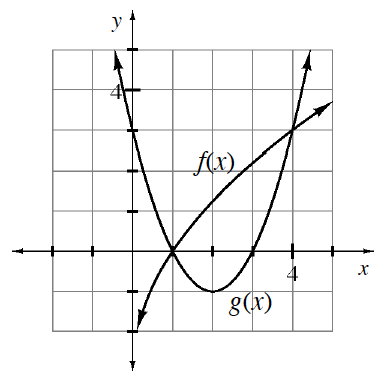### Home > APCALC > Chapter 11 > Lesson 11.2.3 > Problem11-74

11-74.

Multiple Choice: Which integral below calculates the volume of the solid generated when the region bounded by the graphs of $y = f(x)$ and $y = g(x)$ (shown below) is rotated about the $y$-axis?

1. $2 \pi \int _ { 1 } ^ { 4 } x ( f ( x ) - g ( x ) ) d x$

2. $2 \pi \int _ { 1 } ^ { 4 } x ( g ( x ) - f ( x ) ) d x$

3. $\pi \int _ { 1 } ^ { 4 } ( f ( x ) - g ( x ) ) ^ { 2 } d x$

4. $\pi \int _ { 1 } ^ { 4 } ( g ^ { 2 } ( x ) - f ^ { 2 } ( x ) ) d x$

5. None of theseThis is a situation where it works well to use shells. What is the formula for using shells?